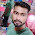### Program 173:Calculate Simple Interest in all Ways

Program 173:             Learn Switch case in detail

```#include<stdio.h>
main()
{
float p,r,t,simpleInterest;
int choice;
printf("Enter\n1 to find out Simple Interest\n2 to find out Principal\n3 to find out rate in % \n4 to find out Time\n");
scanf("%d",&choice);
switch(choice)
{
case 1:
{
printf("Enter Pricipal\n");
scanf("%f",&p);
printf("Enter Rate in percentage \n");
scanf("%f",&r);
printf("Enter Time in years(decimals)\n");
scanf("%f",&t);

simpleInterest=(float)(p*r*t)/100.0;
printf("Simple Interest is %f\n",simpleInterest);
break;
}
case 2:
{
printf("Enter Simple Interest\n");
scanf("%f",&simpleInterest);
printf("Enter Rate in percentage\n");
scanf("%f",&r);
printf("Enter Time in years(decimals)\n");
scanf("%f",&t);
p=(float)(100.0*simpleInterest)/(r*t);
printf("Principal is %f\n",p);
break;

}
case 3:
{
printf("Enter Simple Interest\n");
scanf("%f",&simpleInterest);
printf("Enter Principal\n");
scanf("%f",&p);
printf("Enter Time in years(decimals)\n");
scanf("%f",&t);
r=(float)(100.0*simpleInterest)/(p*t);
printf("Rate is %f%\n",r);
break;
}
case 4:
{
printf("Enter Simple Interest\n");
scanf("%f",&simpleInterest);
printf("Enter Principal\n");
scanf("%f",&p);
printf("Enter Rate in percentage\n");
scanf("%f",&r);
t=(float)((100.0*simpleInterest)/(p*r));
printf("Time is %f years\n",t);
break;
}
}
}

```
Explanation:
Formulae used in Program:
```1.Simple Interest=(Principal*Rate in %* Time in Years)/100

2.Principal=(100*Simple Interest)/(Rate in %* Time in Years)

3.Rate(%)=(100*Simple Interest)/(Principal* Time in Years)

4.Time(Yrs)=(100*Simple Interest)/(Principal* Rate(%)) ```

1. This program starts with initializing :
• p → To store principal in Rupees
• r → To store rate in %
• t → To store time in years
• simpleInterest → To store output
2. ```printf("Enter\n1 to find out Simple Interest\n2 to find out Principal\n
3 to find out rate in % \n4 to find out Time\n");
scanf("%d",&choice);```
To prompt user to choose one of the following as shown in the output.
3. Lets run by giving choice as 2 which is to find out Principal.
4. As the choice is 2. Case 2: will be executed. Learn Switch case in detail
• As per case formula 2 is used for execution
• ``` printf("Enter Simple Interest\n");
scanf("%f",&simpleInterest);```
Taking Simple Interest from user
```    printf("Enter Rate in percentage\n");
scanf("%f",&r);```
Taking Rate from user
```    printf("Enter Time in years(decimals)\n");
scanf("%f",&t);```
Taking Time from user
```    p=(float)(100.0*simpleInterest)/(r*t);
printf("Principal is %f\n",p);
break;```
From formula 2 the above will calculate and stores result in p which is then printed.

Output:

#### 1 comment:

1.factorial hundred In the last few days, the “factorial of 100” is one of the top subjects and a lot of maths geeks compute it using voice assistants such as Alexa, Shiri, etc.

factorial hundred In the last few days, the “factorial of 100” is one of the top subjects and a lot of maths geeks compute it using voice assistants such as Alexa, Shiri, etc.

factorial hundred In the last few days, the “factorial of 100” is one of the top subjects and a lot of maths geeks compute it using voice assistants such as Alexa, Shiri, etc.

 Donate Buy me a coffee \$2.00 USD Buy me a burger \$5.00 USD Buy me a pizza \$10.00 USDCompiler Used by me C-Free 5.0(Recommended) Other Compilers Code Blocks(Recommended) Online Compilers Ideone(Remember to give input before executing online where ever necessary)